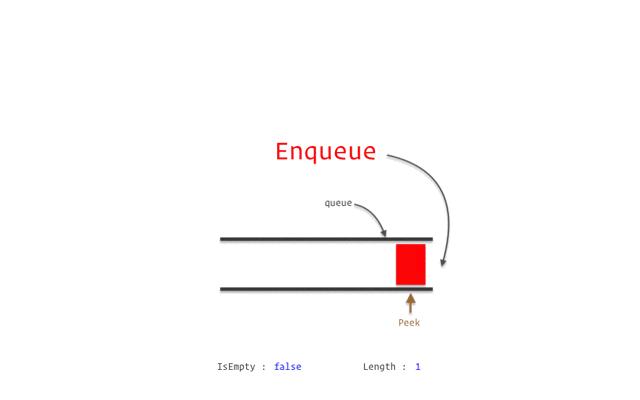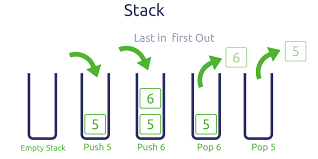# Difference between Array, Queue and Stack

## Introduction

Array, queue, and stack are basic data structures of computer science. Here we will see the comparison between their properties as well as implementation.

## Array

An array is a linear data structure for storing items of the same data type at contiguous memory locations.

Example

Here, 200 is the (base address)starting address assigned to its 1st element, and each element of the array is of integer data type that takes 4 bytes in memory.

• As each element in an array can be accessed by its index, access time is less for the array.

• It is also easier to find the address of any element by using the address of the first element as location of the first element is denoted by the name of the array itself. We need to add a fixed value to it to reach the required element.

Implementation

``````#include<iostream>
using namespace std;

int main()
{
int arr={11,9,17,89,1};

cout<<*arr<<endl;

//accessing  elements  using the address of the first element
for(int i=0;i<5;i++)
{
cout<<*(arr+i)<<" ";
}

return 0;
}``````

Output

## Queue

Queue is also a linear data structure or abstract data type where an element is inserted from one end(i.e., rear), and an element is removed from the opposite end(i.e., front). For such type of property, the queue is called FIFO data structure.

Queue performs mainly two operations—

1. Enqueue to insert an element in the queue from the rear.

2. Dequeue to remove elements from the front.

Examplesource

• For dynamic addition and deletion of elements in a data structure, queues are used. We can achieve our tasks very efficiently as the queue requires O(1) time complexity for all the operations like enqueue and dequeue

• It is used for scheduling tasks in the order of arrival by the operating system.

• To implement many Graph Algorithms like breadth-first search, Dijkstra’s algorithm and Prim’s algorithm queues are used.

Implementation

``````#include<iostream>
using namespace std;
#define SIZE 10
class Queue
{
int a[SIZE];
int rear;
int front;
public:
Queue()
{
rear = front = -1;
}
void enqueue(int x);
int dequeue();
void display();
};

// function for inserting elements to queue
void Queue :: enqueue(int x)
{

if(front == -1) {
front++;
}
if(rear == SIZE-1)
{
cout << "Queue is full";
}
else
{
a[++rear] = x;
cout<<"Element inserted:"<<x<<"\n";
}
}

// function for removeing the element from queue
int Queue :: dequeue()
{
//removes the top element from the queue.
cout<<"Deleted element:"<<a[++front]<<"\n";
}

void Queue :: display()
{
int i;
cout<<"The Remaining elements are:"<<"\n";
for(i = front; i <= rear; i++)
{
cout << a[i] << endl;
}
}

int main()
{
Queue q;
q.enqueue(11);
q.enqueue(13);
q.enqueue(21);
//removes the first element from queue
q.dequeue();
q.display();
return 0;
}``````

## Stack

Stack is also a linear data structure or abstract data type where an element is inserted at the front(i.e, top) and an element is also removed from the same end(i.e.,, top). For such type of property, the stack is called LIFO data structure.

Stack performs mainly two operations
1. push to insert an element at the top.

2. pop to remove elements from the top.

Examplesource

Implementation

``````#include<iostream>
using namespace std;

class Stack
{
int top; // for locating the top of the stack

public:
int a;
Stack()
{
top = -1;
}
void push(int x);
int pop();
void isEmpty();
};

// function for inserting elements to stack
void Stack::push(int x)
{

if(top >= 10)
{
cout << "Stack Overflow......element cannot be inserted\n";
}
else
{
a[++top] = x;
cout << "Inserted element: "<<x<<endl;
}
}

// function for removeing the element from stack
int Stack::pop()
{

if(top < 0)
{
cout << "Stack Underflow--No elements in stack\n";
return 0;
}
else
{
int d = a[top--];
// printing the element which is popped

cout<<"The popped element: "<<d<<"\n";
return d;
}
}

// for checking if the stack empty or not
void Stack::isEmpty()
{
if(top < 0)
{
cout << "Stack is empty \n";
}
else
{
cout << "Stack is not empty \n";
}
}

int main() {
Stack s1;
s1.push(11); //This will push 11 on the stack
s1.push(13); //This will push 13 onto the stack
s1.pop(); //This will pop out the topmost element from stack
}``````

## Difference

Here is the tabular representation of their difference.

## Key Takeaways

This article covered the difference between Array, Queue, and Stack from different perspectives. Having a good hold on these data structures is very important for cracking any coding round.

Check out the CodeStudio library for getting a better hold of the data structures and algorithms.

Side by side, you can also practice a wide variety of coding questions commonly asked in interviews in CodeStudio. Along with coding questions, you can also find the interview experience of scholars working in renowned product-based companies here.

Happy learning!